POLAR EQUATIONSLearning Objectives - This is what you must know after studying the lecture and doing the practice problems!

1.   Change polar equations into rectangular equations.
2.   Change rectangular equations into polar equations.It is often also necessary to transform equations from rectangular to polar form or vice versa.  The following polar-rectangular relationships are still useful in this regard.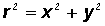(derived from the Pythagorean Theorem. See picture.)(derived from the cosine ratio)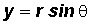(derived from the sine ratio)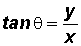(derived from the tangent ratio)

Strategy for Changing Equations in Rectangular Form to Polar Form - see #1 through 4 in the "Examples" document

1. Use one or more of the the conversions,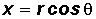,, andto find a polar equation.

2. Write this polar equation as r in terms of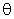. Writing r in therms ofmeans that we must isolate r on one side of the equal sign with coefficient 1.

Strategy for Changing Equations in Polar Form to Rectangular Form - see #5 through 9 in the "Examples" document

1. Use one or more of the conversions,,, andto find a rectangular equation.

2. If a conversion is not readily apparent, you must try one or more of the following:

• Multiply both sides of the equation by r
• Use the Reciprocal or Quotient Identities to change to sines and cosines
• Square both sides of the equation